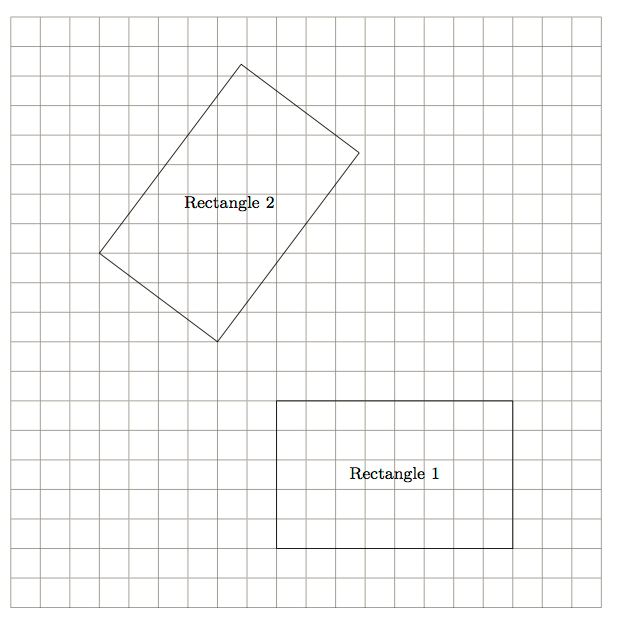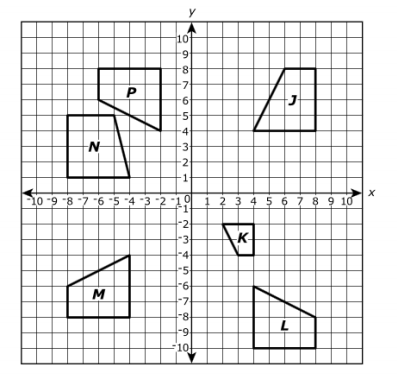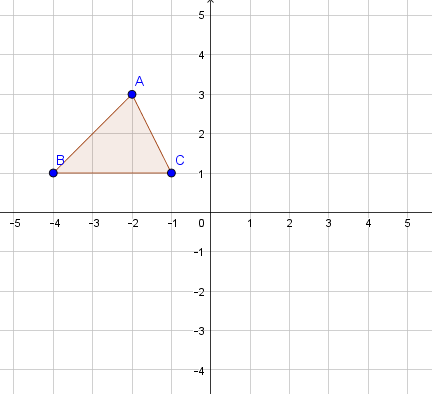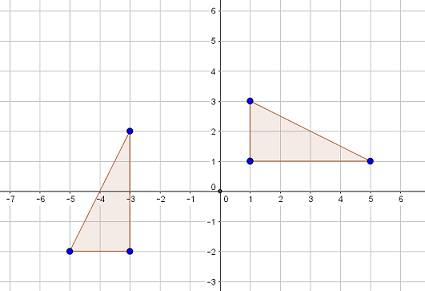# Transformations and Angle Relationships

## Objective

Describe sequences of transformations between figures using rotations and other transformations.

## Common Core Standards

### Core Standards

?

• 8.G.A.1.A — Lines are taken to lines, and line segments to line segments of the same length.

• 8.G.A.1.B — Angles are taken to angles of the same measure.

• 8.G.A.1.C — Parallel lines are taken to parallel lines.

• 8.G.A.2 — Understand that a two-dimensional figure is congruent to another if the second can be obtained from the first by a sequence of rotations, reflections, and translations; given two congruent figures, describe a sequence that exhibits the congruence between them.

?

• 4.MD.C.6

## Criteria for Success

?

1. Perform a combination of transformations, using rotations and other transformations, on a figure.
2. Describe a sequence of transformations with precision, using rotations and other transformations, between two figures (MP.6).
3. Understand that properties of transformations hold true even when there are multiple rigid transformations; for example, the side length of a polygon that is rotated and reflected is the same on the original figure and the transformed figure.

## Tips for Teachers

?

#### Fishtank Plus

• Problem Set
• Student Handout Editor
• Vocabulary Package

## Anchor Problems

?

### Problem 1

Figure 1 is shown in the coordinate plane below.Which figure(s) would Figure 1 map to if it were

• translated?
• reflected?
• rotated?

### Problem 2

Below is a picture of two rectangles with the same length and width:1. Show that the rectangles are congruent by finding a translation followed by a rotation that maps one of the rectangles to the other
2. Explain why the congruence of the two rectangles cannot be shown by translating Rectangle 1 to Rectangle 2.

#### References

Illustrative Mathematics Congruent Rectangles

Congruent Rectangles, accessed on Oct. 13, 2017, 4:01 p.m., is licensed by Illustrative Mathematics under either the CC BY 4.0 or CC BY-NC-SA 4.0. For further information, contact Illustrative Mathematics.

Modified by The Match Foundation, Inc.

### Problem 3

Figures ${J, K, L, M, N,}$ and $P$ are shown on the coordinate plane.Part A:

Which figure can be transformed into Figure P by a translation 2 units to the right followed by a reflection across the $x-$axis?

a.     Figure J

b.     Figure K

c.     Figure L

d.    Figure M

Part B: Which figure can be transformed into Figure L by a 90-degree rotation clockwise about the origin followed by a translation 2 units down?

a.     Figure J

b.     Figure M

c.     Figure N

d.     Figure P

#### References

PARCC Released Items Math Spring Operational 2015 Grade 8 End of Year Released ItemsProblem #10

## Problem Set

?The following resources include problems and activities aligned to the objective of the lesson that can be used to create your own problem set.

• Examples where students perform transformations that involve a combination or rotations and either reflections or translations to determine if two figures are congruent
• Examples where students identify coordinate points of rotated vertices; be sure to include examples of rotations around the origin and around a point on a figure
• Examples where students demonstrate understanding of properties of transformations

?

### Problem 1

Triangle ${{ABC}}$ is rotated ${90^{\circ}}$ clockwise around the origin.Which of the statements below are true? Select all that apply.

a.     Point ${{B'}}$ will be located at ${(1, 4)}$.

b.     Point ${{B'}}$ will be located at ${(4,1)}$.

c.     $\overline{{{B'}}C'}$ will be a vertical line.

d.     $\overline{{{B'}}C'}$ will be parallel to ${\overline{BC}}$.

e.     The area of $A'{{B'}}C'$ will be greater than the area of ${{ABC}}$, but the perimeters will be the same.

f.     Both the areas and perimeters of the original and the rotated figures will be the same.

### Problem 2

Describe how you can use transformations to show that the two figures below are congruent.?